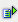The function factdouble (num) calculates the double-factorial of a number.

Syntax: =factdouble(num)Example =factdouble(0) is 0 =factdouble(1) is 1 =factdouble(2) is 2 =factdouble(3) is 3 =factdouble(4) is 8 =factdouble(5) is 15